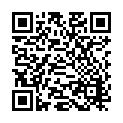Lavoisier S.A.S.
14 rue de Provigny
94236 Cachan cedex
FRANCE

Heures d'ouverture 08h30-12h30/13h30-17h30
Tél.: +33 (0)1 47 40 67 00
Fax: +33 (0)1 47 40 67 02Url canonique : www.lavoisier.fr/livre/autre/the-nature-of-mathematics-and-the-mathematics-of-nature/descriptif_3578362
Url courte ou permalien : www.lavoisier.fr/livre/notice.asp?ouvrage=3578362

# The Nature of Mathematics and the Mathematics of Nature

Langue : Anglais

• ## Sommaire

Chemistry, physics and biology are by their nature genuinely difficult. Mathematics, however, is man-made, and therefore not as complicated. Two ideas form the basis for this book: 1) to use ordinary mathematics to describe the simplicity in the structure of mathematics and 2) to develop new branches of mathematics to describe natural sciences.

Mathematics can be described as the addition, subtraction or multiplication of planes. Using the exponential scale the authors show that the addition of planes gives the polyhedra, or any solid. The substraction of planes gives saddles. The multiplication of planes gives the general saddle equations and the multispirals. The equation of symmetry is derived, which contains the exponential scale with its functions for solids, the complex exponentials with the nodal surfaces, and the GD (Gauss Distribution) mathematics with finite periodicity.

Piece by piece, the authors have found mathematical functions for the geometrical descriptions of chemical structures and the structure building operations. Using the mathematics for dilatation; twins, trillings, fourlings and sixlings are made, and using GD mathematics these are made periodic. This description of a structure is the nature of mathematics itself. Crystal structures and 3D mathematics are synonyms. Mathematics are used to describe rod packings, Olympic rings and defects in solids. Giant molecules such as cubosomes, the DNA double helix, and certain building blocks in protein structures are also described mathematically.

Introduction. References. Publications on 'The Exponential Scale'. The Roots of Mathematics - the Roots of Structure. Multiplication of polynomials. Addition of polynomials. Saddles. Exercises. References. The Natural Function and the Exponential Scale. Polygons and planar geometry. Polyhedra and geometry. Curvature. The fundamental polyhedra - and others. Optimal organisation and higher exponentials. Exercises. References. Periodicity and the Complex Exponential. The translation vector. The complex exponential and some variants. Some other exponentials. Exercises. References. The Screw and the Finite Periodicity with the Circular Punctions. Chirality, the screw and the multi spiral. The bending of a helix. Finite periodicity - molecules and the Larsson cubosomes. Multiplication, Nets and Planar Groups. Lines and saddles. Nets with two planes, and variations. Nets with three planes, and variations. Nets with four planes, and variations. Structures in 3D from the nets. Quasi. Four planes and quasi. Five planes and quasi. The Gauss Distribution Function. The GD function and periodicity. Handmade periodicity. The GD function and periodicity in 3D. The BCC and diamond symmetries. The link to cosine. Handmade Structures and Periodicity. Prelude. Simplest of periodic structures. Contact of spheres in space - structures and surfaces. How tetrahedra and octahedra meet in space. The Rods in Space. Primitive packing of rods. Body centred packing of rods. Tetragonal and hexagonal packing of rods. Larsson cubosomes of rods. Packing of rods, and their related surfaces. The Rings, Addition and Subtraction. Some simple examples of subtraction and addition in 3D. The rings. More ways to make rings. More subtraction - hyperbolic polyhedra. Periodic Dilation - Concentric Symmetry. Dilatation and translation in 2D. Dilatation and translation in 3D. Pure dilatation. Appendices. Subject Index.

Date de parution :

Ouvrage de 354 p.

Sous réserve de disponibilité chez l'éditeur.

Prix indicatif 307,55 €

### Thème de The Nature of Mathematics and the Mathematics of Nature :

En continuant à naviguer, vous autorisez Lavoisier à déposer des cookies à des fins de mesure d'audience. Pour en savoir plus et paramétrer les cookies, rendez-vous sur la page Confidentialité & Sécurité.
FERMER